# Fitting Multiple Orders of HRC-S/LETG DataSherpa Threads (CIAO 4.13 Sherpa v1)

## Overview

#### Synopsis:

Because of the low energy resolution in the HRC-S, the PHA2 file contains two rows (negative and postive) containing all the spectral orders. While it is not possible to separate the overlapping orders, they can be modeled in Sherpa by defining the instrument response as a composite of the orders in which you are interested.

This thread uses response files (gRMFs and gARFs) created with CIAO to model and fit the first three positive and negative orders of the spectra.

Last Update: 21 Dec 2020 - Updated for CIAO 4.13: use new plot functionality and remove the CIAO 4.12 work arounds.

## Getting Started

unix% download_chandra_obsid 460

The files used in this example were created by following several of the CIAO Grating threads:

Here is a list of all the necessary files:

spectra:
460_leg_m1_bin10.pha
460_leg_p1_bin10.pha

rmfs:
460_leg_-1.grmf
460_leg_-2.grmf
460_leg_-3.grmf
460_leg_1.grmf
460_leg_2.grmf
460_leg_3.grmf

arfs:
460_LEG_-1.garf
460_LEG_-2.garf
460_LEG_-3.garf
460_LEG_1.garf
460_LEG_2.garf
460_LEG_3.garf


The spectra that will be used in this session have already been binned by a factor of 10. The data are input to Sherpa with the load_pha command (a subset of the load_data command):

sherpa> load_pha(1, "460_leg_m1_bin10.pha")
statistical errors were found in file '460_leg_m1_bin10.pha'
but not used; to use them, re-read with use_errors=True
read background_up into a dataset from file 460_leg_m1_bin10.pha
read background_down into a dataset from file 460_leg_m1_bin10.pha

statistical errors were found in file '460_leg_p1_bin10.pha'
but not used; to use them, re-read with use_errors=True
read background_up into a dataset from file 460_leg_p1_bin10.pha
read background_down into a dataset from file 460_leg_p1_bin10.pha


Sherpa now refers to the negative-order spectrum as data set 1 and the positive-order spectrum as data set 2.

To establish the instrument response, the individual instrument response files (gRMFs and gARFs) need to be read into Sherpa for each of the six spectral orders (+/- 1,2,3). If the ARF and RMF filenames are recorded in the header of the PHA file, Sherpa will load them automatically; if not, they need to be loaded manually with the load_arf/load_rmf or load_multi_arfs/load_multi_rmfs commands.

sherpa> load_multi_arfs(1, ["460_LEG_-1.garf","460_LEG_-2.garf","460_LEG_-3.garf"], [1,2,3])



OR

sherpa> for num in range(1,4):

sherpa> for num in range(1,4):


OR

sherpa> arf_ids = range(1, 4)
sherpa> rmf_ids = arf_ids[:]

sherpa> rmfs_1 = [f"460_leg_-{id}.grmf" for id in rmf_ids]
sherpa> arfs_1 = [f"460_LEG_-{id}.garf" for id in arf_ids]

sherpa> rmfs_2 = [f"460_leg_{id}.grmf" for id in rmf_ids]
sherpa> arfs_2 = [f"460_LEG_{id}.garf" for id in arf_ids]



We may list the data set and response IDs established in the Sherpa session with the list_data_ids and list_response_ids commands. For viewing detailed information about all loaded data sets and associated ARF and RMF responses, the show_all, show_data, and show_bkg commands are available (which provide the option to save the output data to a file with the 'outfile' argument) following list commands or with get_data, and return information about each ARF and RMF with the get_arf and get_rmf commands.

sherpa> list_data_ids()
[1, 2]

sherpa> list_response_ids()
[1, 2, 3]

sherpa> show_data()
Data Set: 1
Filter: 0.0603-12.1032 Energy (keV)
Bkg Scale: 0.2
Noticed Channels: 1-16384
name           = 460_leg_m1_bin10.pha
channel        = Float64
counts         = Float64
staterror      = None
syserror       = None
bin_lo         = Float64
bin_hi         = Float64
grouping       = Int16
quality        = Int16
exposure       = 39939.2745006
backscal       = 1.0
areascal       = 1.0
grouped        = True
subtracted     = False
units          = energy
rate           = True
plot_fac       = 0
response_ids   = [1, 2, 3]
background_ids = [1, 2]

RMF Data Set: 1:1
name     = 460_leg_-1.grmf
detchans = 16384
energ_lo = Float64
energ_hi = Float64
n_grp    = UInt64
f_chan   = UInt32
n_chan   = UInt32
matrix   = Float64
offset   = 1
e_min    = Float64
e_max    = Float64
ethresh  = 1e-10

ARF Data Set: 1:1
name     = 460_LEG_-1.garf
energ_lo = Float64
energ_hi = Float64
specresp = Float64
bin_lo   = Float64
bin_hi   = Float64
exposure = 39910.3861311
ethresh  = 1e-10

RMF Data Set: 1:2
name     = 460_leg_-2.grmf
detchans = 16384
energ_lo = Float64
energ_hi = Float64
n_grp    = UInt64
f_chan   = UInt32
n_chan   = UInt32
matrix   = Float64
offset   = 1
e_min    = Float64
e_max    = Float64
ethresh  = 1e-10

ARF Data Set: 1:2
name     = 460_LEG_-2.garf
energ_lo = Float64
energ_hi = Float64
specresp = Float64
bin_lo   = Float64
bin_hi   = Float64
exposure = 39910.3861311
ethresh  = 1e-10

RMF Data Set: 1:3
name     = 460_leg_-3.grmf
detchans = 16384
energ_lo = Float64
energ_hi = Float64
n_grp    = UInt64
f_chan   = UInt32
n_chan   = UInt32
matrix   = Float64
offset   = 1
e_min    = Float64
e_max    = Float64
ethresh  = 1e-10

ARF Data Set: 1:3
name     = 460_LEG_-3.garf
energ_lo = Float64
energ_hi = Float64
specresp = Float64
bin_lo   = Float64
bin_hi   = Float64
exposure = 39910.3861311
ethresh  = 1e-10

Background Data Set: 1:1
Filter: 0.0603-12.1032 Energy (keV)
Noticed Channels: 1-16384
name           = 460_leg_m1_bin10.pha
channel        = Float64
counts         = Float64
staterror      = None
syserror       = None
bin_lo         = Float64
bin_hi         = Float64
grouping       = Int16
quality        = Int16
exposure       = 39939.2745006
backscal       = 5.0
areascal       = None
grouped        = True
subtracted     = False
units          = energy
rate           = True
plot_fac       = 0
response_ids   = []
background_ids = []

Background Data Set: 1:2
Filter: 0.0603-12.1032 Energy (keV)
Noticed Channels: 1-16384
name           = 460_leg_m1_bin10.pha
channel        = Float64
counts         = Float64
staterror      = None
syserror       = None
bin_lo         = Float64
bin_hi         = Float64
grouping       = Int16
quality        = Int16
exposure       = 39939.2745006
backscal       = 5.0
areascal       = None
grouped        = True
subtracted     = False
units          = energy
rate           = True
plot_fac       = 0
response_ids   = []
background_ids = []

Data Set: 2
Filter: 0.0603-12.1032 Energy (keV)
Bkg Scale: 0.2
Noticed Channels: 1-16384
name           = 460_leg_p1_bin10.pha
channel        = Float64
counts         = Float64
staterror      = None
syserror       = None
bin_lo         = Float64
bin_hi         = Float64
grouping       = Int16
quality        = Int16
exposure       = 39939.2745006
backscal       = 1.0
areascal       = 1.0
grouped        = True
subtracted     = False
units          = energy
rate           = True
plot_fac       = 0
response_ids   = [1, 2, 3]
background_ids = [1, 2]

RMF Data Set: 2:1
name     = 460_leg_1.grmf
detchans = 16384
energ_lo = Float64
energ_hi = Float64
n_grp    = UInt64
f_chan   = UInt32
n_chan   = UInt32
matrix   = Float64
offset   = 1
e_min    = Float64
e_max    = Float64
ethresh  = 1e-10

ARF Data Set: 2:1
name     = 460_LEG_1.garf
energ_lo = Float64
energ_hi = Float64
specresp = Float64
bin_lo   = Float64
bin_hi   = Float64
exposure = 39910.3861311
ethresh  = 1e-10

RMF Data Set: 2:2
name     = 460_leg_2.grmf
detchans = 16384
energ_lo = Float64
energ_hi = Float64
n_grp    = UInt64
f_chan   = UInt32
n_chan   = UInt32
matrix   = Float64
offset   = 1
e_min    = Float64
e_max    = Float64
ethresh  = 1e-10

ARF Data Set: 2:2
name     = 460_LEG_2.garf
energ_lo = Float64
energ_hi = Float64
specresp = Float64
bin_lo   = Float64
bin_hi   = Float64
exposure = 39910.3861311
ethresh  = 1e-10

RMF Data Set: 2:3
name     = 460_leg_3.grmf
detchans = 16384
energ_lo = Float64
energ_hi = Float64
n_grp    = UInt64
f_chan   = UInt32
n_chan   = UInt32
matrix   = Float64
offset   = 1
e_min    = Float64
e_max    = Float64
ethresh  = 1e-10

ARF Data Set: 2:3
name     = 460_LEG_3.garf
energ_lo = Float64
energ_hi = Float64
specresp = Float64
bin_lo   = Float64
bin_hi   = Float64
exposure = 39910.3861311
ethresh  = 1e-10

Background Data Set: 2:1
Filter: 0.0603-12.1032 Energy (keV)
Noticed Channels: 1-16384
name           = 460_leg_p1_bin10.pha
channel        = Float64
counts         = Float64
staterror      = None
syserror       = None
bin_lo         = Float64
bin_hi         = Float64
grouping       = Int16
quality        = Int16
exposure       = 39939.2745006
backscal       = 5.0
areascal       = None
grouped        = True
subtracted     = False
units          = energy
rate           = True
plot_fac       = 0
response_ids   = []
background_ids = []

Background Data Set: 2:2
Filter: 0.0603-12.1032 Energy (keV)
Noticed Channels: 1-16384
name           = 460_leg_p1_bin10.pha
channel        = Float64
counts         = Float64
staterror      = None
syserror       = None
bin_lo         = Float64
bin_hi         = Float64
grouping       = Int16
quality        = Int16
exposure       = 39939.2745006
backscal       = 5.0
areascal       = None
grouped        = True
subtracted     = False
units          = energy
rate           = True
plot_fac       = 0
response_ids   = []
background_ids = []


The response orders are stored in the order in which they are loaded, noted by the 'resp_id' value. The first response in the list of response IDs is treated as the primary (usually resp_id=1), i.e. it is used as the mapping from wavelength or energy units to spectral channel. The primary response is used as the independent variable grid for plotting, as well as for translating the y-axis to the proper units (keV or Angstroms). During fitting, the calculation of the source model uses the respective ARF grid from each of the responses. Similarly, the respective response is used when folding through the response matrix.

## Plotting Orders

Before plotting the data with the plot command, ensure that the units field of each data set is set to "wavelength" with the set_analysis command, as in the following example for data set 1:

sherpa> set_analysis("wave")
sherpa> get_analysis()
'wavelength'


The data may now be plotted:

sherpa> plot("data", 1, "data", 2)


Figure 1 shows the resulting plot.

### Figure 1: Plotting the two orders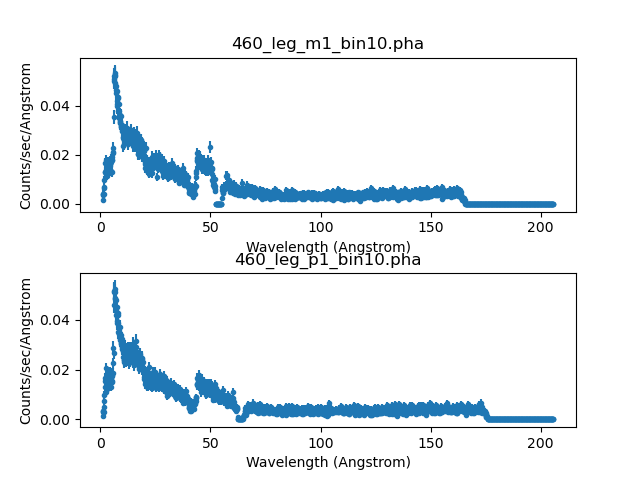### Figure 1: Plotting the two orders

Plot of HRC LEG +/- 1 orders for 3C 273

We can use Matplotlib to adjust the features of the plot, such as setting colors, changing font styles, and repositioning objects. Below, get_data_plot_prefs is used to to connect the data points with a solid line, and to remove data point symbols and y-axis error bars from the plot. The resulting plot is displayed in Figure 2.

sherpa> prefs = get_data_plot_prefs()
sherpa> prefs["linestyle"] = "solid"
sherpa> prefs["marker"] = "None"
sherpa> prefs["yerrorbars"] = False
sherpa> plot('data', 1, 'data', 2)


### Figure 2: Modifying the plot of orders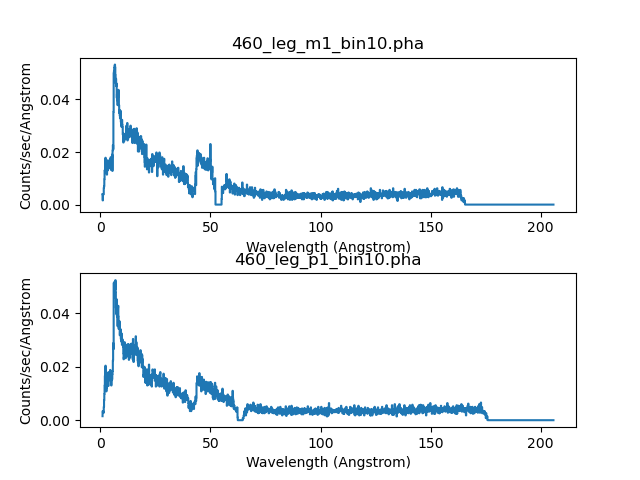### Figure 2: Modifying the plot of orders

Plot of HRC LEG +/- 1 orders after changing the plot defaults.

## Filtering the Data

There are two plate gaps in the HRC-S detector: one at ~50 Å and the other at ~60 Å; see Figure 7.1 in the POG for the HRC-S detector layout. The effect of dithering into these gaps appear in negative-order and positive-order data, respectively, as a flat area of zero counts. The regions where the data are in a plate gap are evident in Figure 3.

sherpa> plot_data(1)
sherpa> plot_data(2, overplot=True)
sherpa> plt.xlim(40, 75)
sherpa> plt.ylim(-0.001, 0.022)


### Figure 3: Regions where the data dithered into a plate gap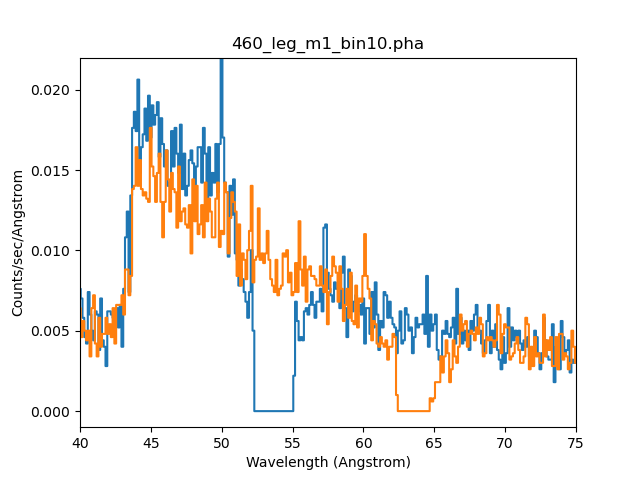### Figure 3: Regions where the data dithered into a plate gap

There are two plate gaps in the HRC-S detector: one at ~50 Å and the other at ~60 Å. The effect of dithering into these gaps can be seen in the negative-order (blue line) and positive-order data (orange line).

These regions are ignored in the fitting so that the statistic calculations are not skewed:

sherpa> ignore_id(1, 49., 56.)
sherpa> ignore_id(2, 59., 66.)


You may wish to adjust the limits to exclude more or less data around this region. Any other desired filters may be applied to the data at this point as well. In this case we are going to ignore some low and high wavelength data for both data sets:

sherpa> ignore(None, 1.5)
sherpa> ignore(155, None)


The combined dataset looks like Figure 4:

sherpa> plot_data(1, alpha=0.5)
sherpa> plot_data(2, alpha=0.5, overplot=True)


### Figure 4: Overlying the two datasets to be fit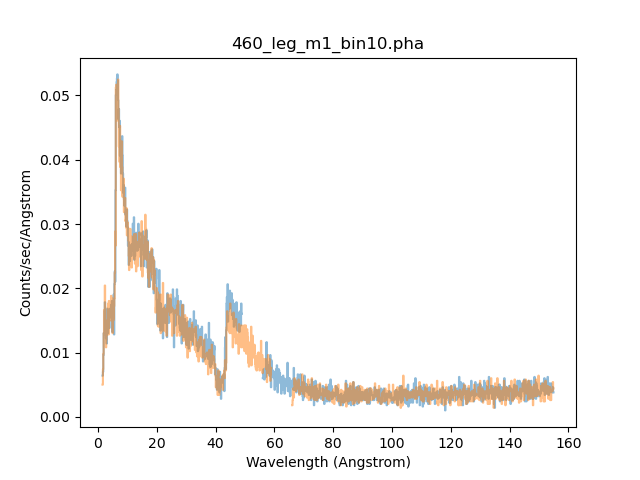### Figure 4: Overlying the two datasets to be fit

New in CIAO 4.13 is the ability to set the transparency of the plot items with the alpha option, which goes from 0 (fully transparent) to 1 (fully opaque).

## Defining the Source Model

We plan on subtracting the background from the data (rather than fitting it simultaneously with the source data), so it is only necessary to create a source model expression. We model this source with a broken power-law (xsbknpower) absorbed by the interstellar medium (xsphabs).

In this example, we choose to use the XSpec version of the models. These models are defined such that the x-values are always interpreted as energy bins. When the analysis setting is using non-energy bins (e.g., WAVE in this case) and an XSpec model is defined, Sherpa converts the bins to energy before sending them to the XSpec model. After the XSpec model finishes, Sherpa converts back to the original units. Sherpa also scales the model values appropriately (e.g., if counts/keV came out of the XSpec model, and Sherpa is working with wavelength bins, then Sherpa scales the output of the XSpec model to counts/Angstrom).

The absorption model will be referred to as abs1, and the broken power-law will be bpow1; the product of the two is assigned as the source model for the data sets:

sherpa> mdl = xsphabs.abs1 * xsbknpower.bpow1
sherpa> set_source(mdl)
sherpa> set_source(2, mdl)

sherpa> show_model()
Model: 1
(39939.274500594 * MultiResponseSumModel(apply_rmf(apply_arf(((xsphabs.abs1 * xsbknpower.bpow1)),apply_rmf(apply_arf(((xsphabs.abs1 * xsbknpower.bpow1)),apply_rmf(apply_arf(((xsphabs.abs1 * xsbknpower.bpow1))))
Param        Type          Value          Min          Max      Units
-----        ----          -----          ---          ---      -----
abs1.nH      thawed            1            0       100000 10^22 atoms / cm^2
bpow1.PhoIndx1 thawed            1           -2            9
bpow1.BreakE thawed            5         0.01        1e+06        keV
bpow1.PhoIndx2 thawed            2           -2            9
bpow1.norm   thawed            1            0        1e+24

Model: 2
(39939.274500594 * MultiResponseSumModel(apply_rmf(apply_arf(((xsphabs.abs1 * xsbknpower.bpow1)),apply_rmf(apply_arf(((xsphabs.abs1 * xsbknpower.bpow1)),apply_rmf(apply_arf(((xsphabs.abs1 * xsbknpower.bpow1))))
Param        Type          Value          Min          Max      Units
-----        ----          -----          ---          ---      -----
abs1.nH      thawed            1            0       100000 10^22 atoms / cm^2
bpow1.PhoIndx1 thawed            1           -2            9
bpow1.BreakE thawed            5         0.01        1e+06        keV
bpow1.PhoIndx2 thawed            2           -2            9
bpow1.norm   thawed            1            0        1e+24

sherpa> abs1.nh = 0.1
sherpa> bpow1.norm = 0.0434012

sherpa> show_model()
Model: 1
(39939.274500594 * MultiResponseSumModel(apply_rmf(apply_arf(((xsphabs.abs1 * xsbknpower.bpow1)),apply_rmf(apply_arf(((xsphabs.abs1 * xsbknpower.bpow1)),apply_rmf(apply_arf(((xsphabs.abs1 * xsbknpower.bpow1))))
Param        Type          Value          Min          Max      Units
-----        ----          -----          ---          ---      -----
abs1.nH      thawed          0.1            0       100000 10^22 atoms / cm^2
bpow1.PhoIndx1 thawed            1           -2            9
bpow1.BreakE thawed            5         0.01        1e+06        keV
bpow1.PhoIndx2 thawed            2           -2            9
bpow1.norm   thawed    0.0434012            0        1e+24

Model: 2
(39939.274500594 * MultiResponseSumModel(apply_rmf(apply_arf(((xsphabs.abs1 * xsbknpower.bpow1)),apply_rmf(apply_arf(((xsphabs.abs1 * xsbknpower.bpow1)),apply_rmf(apply_arf(((xsphabs.abs1 * xsbknpower.bpow1))))
Param        Type          Value          Min          Max      Units
-----        ----          -----          ---          ---      -----
abs1.nH      thawed          0.1            0       100000 10^22 atoms / cm^2
bpow1.PhoIndx1 thawed            1           -2            9
bpow1.BreakE thawed            5         0.01        1e+06        keV
bpow1.PhoIndx2 thawed            2           -2            9
bpow1.norm   thawed    0.0434012            0        1e+24


Note that if we had not set initial parameter values for the model, we could have used the guess Sherpa function to estimate the initial parameter values for each model component separately, based on the data input to the session. To have Sherpa automatically query for initial parameter values when a model is established, set 'paramprompt(True)' (it is 'False' by default).

We continue to modify a few of the initial parameter values:

sherpa> abs1.nh = 1.81e-02
sherpa> freeze(abs1.nh)

sherpa> bpow1.breake = 1


We choose to set the break energy [keV] to a lower starting point, and the hydrogen column density (nH) is set to the Galactic value and then frozen (which means it will not be allowed to vary during the fit). The rest of the parameters remain thawed.

## Examining Method & Statistic Settings

Next we check the current method and statistics settings:

sherpa> show_method()
Optimization Method: LevMar
name    = levmar
ftol    = 1.19209289551e-07
xtol    = 1.19209289551e-07
gtol    = 1.19209289551e-07
maxfev  = None
epsfcn  = 1.19209289551e-07
factor  = 100.0
verbose = 0

sherpa> show_stat()
Statistic: Chi2Gehrels
Chi Squared with Gehrels variance.

The variance is estimated from the number of counts in each bin,
but unlike Chi2DataVar, the Gaussian approximation is not
used. This makes it more-suitable for use with low-count data.

The standard deviation for each bin is calculated using the
approximation from _:

sigma(i,S) = 1 + sqrt(N(i,s) + 0.75)

where the higher-order terms have been dropped. This is accurate
to approximately one percent. For data where the background has
not been subtracted then the error term is:

sigma(i) = sigma(i,S)

whereas with background subtraction,

sigma(i)^2 = sigma(i,S)^2 + [A(S)/A(B)]^2 sigma(i,B)^2

A(B) is the off-source "area", which could be
the size of the region from which the background is extracted, or
the length of a background time segment, or a product of the two,
etc.; and A(S) is the on-source "area". These terms may be defined
for a particular type of data: for example, PHA data sets A(B) to
BACKSCAL * EXPOSURE from the background data set and A(S) to
BACKSCAL * EXPOSURE from the source data set.

--------
Chi2DataVar, Chi2ModVar, Chi2XspecVar

Notes
-----
The accuracy of the error term when the background has been
subtracted has not been determined. A preferable approach to
background subtraction is to model the background as well as the
source signal.

References
----------

..  "Confidence limits for small numbers of events in
astrophysical data", Gehrels, N. 1986, ApJ, vol 303,
p. 336-346.

sherpa> set_method_opt("finalsimplex", 0)

sherpa> set_stat("chi2datavar")


For this fit, we decide to change the statistic setting from the default (chi2gehrels) to chi2datavar, and the fitting optimization method from LevMar to Nelder-Mead. For a list of all the available methods and statistic settings with explanations, see the Sherpa pages Optimization Methods and Statistcs. To change the current method and statistic, use set_method and set_stat.

## Subtracting the Background and Grouping the Data

The final thing to do before fitting is perform background subtraction on the data.

sherpa> subtract()
sherpa> subtract(2)


## Fitting

The data sets are now simultaneously fit:

sherpa> fit()
Datasets              = 1, 2
Statistic             = chi2datavar
Initial fit statistic = 15900.5
Final fit statistic   = 2453.98 at function evaluation 290
Data points           = 2340
Degrees of freedom    = 2336
Probability [Q-value] = 0.0438601
Reduced statistic     = 1.05051
Change in statistic   = 13446.5
bpow1.PhoIndx1   2.19451
bpow1.BreakE   0.749034
bpow1.PhoIndx2   1.61622
bpow1.norm     0.019906


To plot the fits:

sherpa> plot("fit", 1, "fit", 2)

sherpa> plt.suptitle("3C 273 (ObsID 460)")

sherpa> plt.sca(plt.gcf().axes)
sherpa> plt.title("")
sherpa> plt.xlabel("")
sherpa> plt.ylabel(r"Counts/sec/$\AA$")
sherpa> plt.text(x=125, y=0.04, s="LEG, -1 order",color="green")

sherpa> plt.sca(plt.gcf().axes)
sherpa> plt.title("")
sherpa> plt.xlabel("")
sherpa> plt.ylabel(r"Counts/sec/$\AA$")
sherpa> plt.text(x=125, y=0.04, s="LEG, +1 order",color="green")


The Matplotlib pyplot commands are used to add labels to the drawing areas. The plot is shown in Figure 5.

### Figure 5: Fit to the LEG +/- 1,2,3 orders of ObsID 460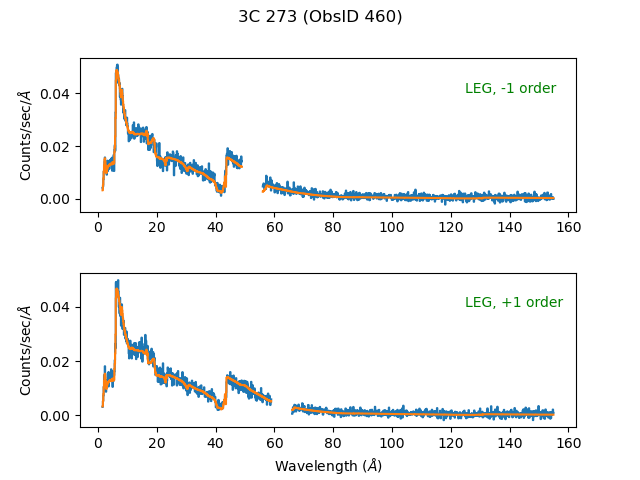### Figure 5: Fit to the LEG +/- 1,2,3 orders of ObsID 460

Plot of the model fit to the spectral orders, with plot title and labels added.

Notice that Sherpa does not attempt to fit the regions that we chose to omit.

sherpa>  show_filter()
Data Set Filter: 1
1.6101-48.8624,56.1124-154.8625 Wavelength (Angstrom)

Data Set Filter: 2
1.6101-58.8624,66.1124-154.8625 Wavelength (Angstrom)


By omitting the regions of data over a plate gap, the residuals are contained within approximately 3σ. This will improve the statistical calculations shown in the Examining Fit Results section.

It is also useful to plot the fit with the residuals (Figure 6):

sherpa> plot_fit_delchi(alpha=0.5)
sherpa> plot_fit_delchi(2, alpha=0.5, overplot=True)
sherpa> plt.title("")


### Figure 6: Fit and residuals for the negative-order spectrum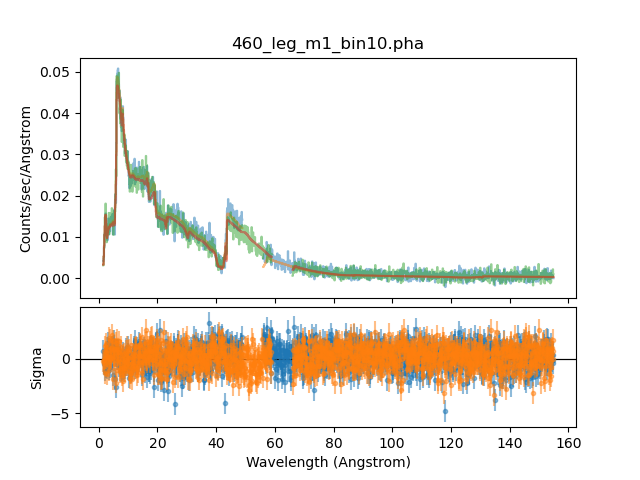### Figure 6: Fit and residuals for the negative-order spectrum

New in CIAO 4.13 is the ability to use overplot with the two-plot routines like plot_fit_resid and plot_bkg_fit_ratio.

Finally, we may use the plot_order and plot_model commands to plot spectral orders individually or simultaneously. For example, we can compare the combined model with the three orders with:

sherpa> plot_model(ylog=True)
sherpa> plt.gca().lines[-2].set_label("All")

sherpa> plot_order(1, 1, alpha=0.8, color='darkred', overplot=True)
sherpa> plt.gca().lines[-2].set_label(r"$1^{st}$")

sherpa> plot_order(1, 2, alpha=0.8, color='pink', overplot=True)
sherpa> plt.gca().lines[-2].set_label(r"$2^{nd}$")

sherpa> plot_order(1, 3, alpha=0.8, color='orange', overplot=True)
sherpa> plt.gca().lines[-2].set_label(r"$3^{rd}$")

sherpa> plt.title("Model Orders")
sherpa> plt.legend(loc="upper right")

sherpa> plt.ylabel(r"$\mathrm{normalized\ counts}\ \mathrm{sec^{-1}}\ \mathrm{\AA^{-1}}$")
sherpa> plt.xlabel(r"$m\lambda [\AA]$")

sherpa> plt.ylim(1e-5, 1e-1)


### Figure 7: Plotting a combination of orders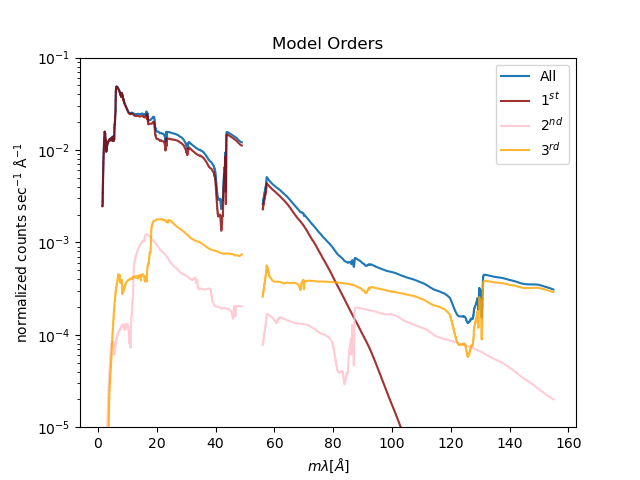### Figure 7: Plotting a combination of orders

Plot of negative spectral orders, with the convolved model contributions from orders 1, 2, and 3 shown in dark red, pink, and orange, respectively.

## Examining Fit Results

The χ2 goodness-of-fit information is reported with the best-fit values after a fit is performed; and the get_fit_results and show_fit commands allow subsequent access to this information post-fitting:

sherpa> show_fit()
name         = simplex
ftol         = 1.19209289551e-07
maxfev       = None
initsimplex  = 0
finalsimplex = 0
step         = None
verbose      = 0

Statistic: Chi2DataVar
Chi Squared with data variance.

The variance in each bin is estimated from the data value in that
bin.

If the number of counts in each bin is large, then the shape of
the Poisson distribution from which the counts are sampled tends
asymptotically towards that of a Gaussian distribution, with
variance

sigma(i)^2 = N(i,S) + [A(S)/A(B)]^2 N(i,B)

where N is the number of on-source (and off-source) bins included
in the fit. The background term appears only if an estimate of the
background has been subtracted from the data.

A(B) is the off-source "area", which could be
the size of the region from which the background is extracted, or
the length of a background time segment, or a product of the two,
etc.; and A(S) is the on-source "area". These terms may be defined
for a particular type of data: for example, PHA data sets A(B) to
BACKSCAL * EXPOSURE from the background data set and A(S) to
BACKSCAL * EXPOSURE from the source data set.

--------
Chi2Gehrels, Chi2ModVar, Chi2XspecVar

Datasets              = 1, 2
Statistic             = chi2datavar
Initial fit statistic = 15900.5
Final fit statistic   = 2453.98 at function evaluation 290
Data points           = 2340
Degrees of freedom    = 2336
Probability [Q-value] = 0.0438601
Reduced statistic     = 1.05051
Change in statistic   = 13446.5
bpow1.PhoIndx1   2.19451
bpow1.BreakE   0.749034
bpow1.PhoIndx2   1.61622
bpow1.norm     0.019906


The number of bins in the fit (Data points), the number of degrees of freedom (i.e. the number of bins minus the number of free parameters), and the final fit statistic value are reported. If the chosen statistic is one of the χ2 statistics, as in this example, the reduced statistic, i.e. the statistic value divided by the number of degrees of freedom, and the probability (Q-value), are included as well.

The calc_chisqr command calculates the statistic contribution per bin; in this example, the results for data set 1 are returned (here restricted to the first ten elements):

sherpa> print(calc_chisqr()[0:10])
[0.14570656 0.10875353 0.00318566 0.04441808 0.10672288 0.0187317
2.0435877  0.13838234 0.1227366  0.42597032]


The covar (covariance) command can be used to estimate confidence intervals for the thawed parameters—though this method may not constrain parameters where the parameter space is not smooth. In this case, we can try the confidence method (conf):

sherpa> covar()
Datasets              = 1, 2
Confidence Method     = covariance
Iterative Fit Method  = None
Statistic             = chi2datavar
covariance 1-sigma (68.2689%) bounds:
Param            Best-Fit  Lower Bound  Upper Bound
-----            --------  -----------  -----------
bpow1.PhoIndx1      2.19451   -0.0158858    0.0158858
bpow1.BreakE     0.749034   -0.0189187    0.0189187
bpow1.PhoIndx2      1.61622   -0.0134631    0.0134631
bpow1.norm       0.019906 -0.000311853  0.000311853

sherpa> set_conf_opt("fast", False)   # to force conf() to use current method, by default set to "False"
sherpa> conf()
bpow1.PhoIndx1 lower bound:     -0.0208864
bpow1.PhoIndx2 lower bound:     -0.0162156
bpow1.BreakE lower bound:       -0.0198805
bpow1.PhoIndx1 upper bound:     0.0153508
bpow1.PhoIndx2 upper bound:     0.0128024
bpow1.BreakE upper bound:       0.0359257
bpow1.norm lower bound: -0.000309416
bpow1.norm upper bound: 0.000448288
Datasets              = 1, 2
Confidence Method     = confidence
Iterative Fit Method  = None
Statistic             = chi2datavar
confidence 1-sigma (68.2689%) bounds:
Param            Best-Fit  Lower Bound  Upper Bound
-----            --------  -----------  -----------
bpow1.PhoIndx1      2.19451   -0.0208864    0.0153508
bpow1.BreakE     0.749034   -0.0198805    0.0359257
bpow1.PhoIndx2      1.61622   -0.0162156    0.0128024
bpow1.norm       0.019906 -0.000309416  0.000448288


## Saving and Quitting the Session

Before exiting Sherpa, you may wish to save the session in order to return to the analysis at a later point:

sherpa> save("460_fitting_session.save")


All the information about the current session is written to 460_fitting_session.save, a binary file. It may be loaded into Sherpa again with the restore command.

Finally, quit the session:

sherpa> quit


## Scripting It

The file fit.py is a Python script which performs the primary commands used above; it can be executed by typing %run -i fit.py on the Sherpa command line.

The Sherpa script command may be used to save everything typed on the command line in a Sherpa session:

sherpa> script(filename="sherpa.log", clobber=False)


(Note that restoring a Sherpa session from such a file could be problematic since it may include syntax errors, unwanted fitting trials, et cetera.)

## History

 06 Aug 2008 updated for CIAO 4.1 04 Dec 2008 plot_order() is available in Sherpa 4.1 13 Dec 2008 neldermead method used in place of levmar 29 Apr 2009 new script command is available with CIAO 4.1.2 10 Jan 2010 updated for CIAO 4.2 13 Jul 2010 updated for CIAO 4.2 Sherpa v2: removal of S-Lang version of thread. 22 Jan 2012 reviewed for CIAO 4.4 (no changes) 13 Dec 2012 reviewed for CIAO 4.5: group commands no longer clear the existing data filter 03 Mar 2014 reviewed for CIAO 4.6: no changes 26 Feb 2015 updated for CIAO 4.7: no content change 14 Dec 2015 updated for CIAO 4.8, removed references to CIAO 3.4. 14 Nov 2016 reviewed for CIAO 4.9; no content change, updated fit results. 30 May 2018 reviewed for CIAO 4.10, no content change 11 Dec 2018 reviewed for CIAO 4.11, no content change 13 Dec 2019 replaced ChIPS commands and figures with Matplotlib equivalent. 21 Dec 2020 Updated for CIAO 4.13: use new plot functionality and remove the CIAO 4.12 work arounds.